### IMO Shortlist 2006 problem A3

Kvaliteta:
Avg: 0,0
Težina:
Avg: 7,0
The sequence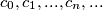$c_{0}, c_{1}, . . . , c_{n}, . . .$ is defined by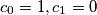$c_{0}= 1, c_{1}= 0$, and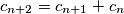$c_{n+2}= c_{n+1}+c_{n}$ for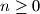$n \geq 0$. Consider the set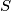$S$ of ordered pairs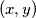$(x, y)$ for which there is a finite set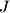$J$ of positive integers such that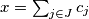$x=\sum_{j \in J}{c_{j}}$,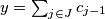$y=\sum_{j \in J}{c_{j-1}}$. Prove that there exist real numbers$\alpha$,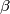$\beta$, and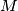$M$ with the following property: An ordered pair of nonnegative integers$(x, y)$ satisfies the inequality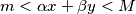$m < \alpha x+\beta y < M$ if and only if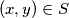$(x, y) \in S$.

Remark: A sum over the elements of the empty set is assumed to be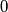$0$.
Izvor: Međunarodna matematička olimpijada, shortlist 2006